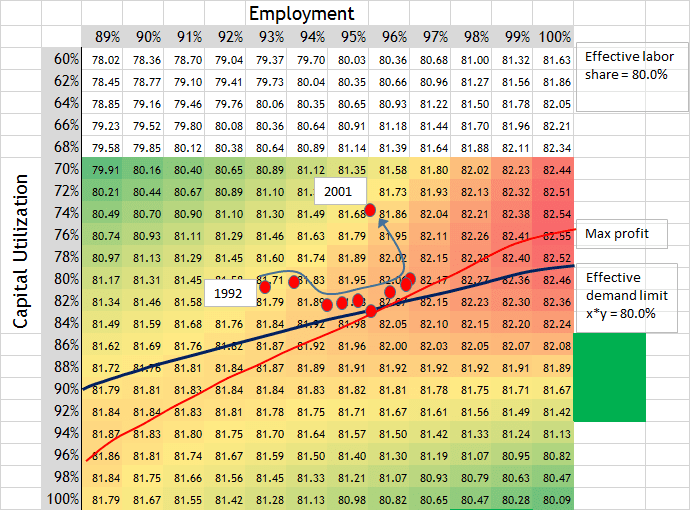## Update to Cobra Equation… 2001 recession

In the morning I posted about the Cobra equation and included some graphs using past data. The graphs showed how capital utilization and employment of labor would increase up to the profit maximization line and then fall back into a recession. But I did not include a graph for the years before the 2001 recession, because it is a special case. So I present it now.

The red dots are from the 3rd quarter for each year, from 1992 to 2001. The recession started in 2001. The coefficient a is 0.6652. (see below for equation.) The effective labor share was 80%. The effective demand limit line shows (capital utilization * employment = 80%) The red line is the measure of profitability. (See equation below) As the numbers in the graph increase, profitability increases. The red line shows the maximum profitability for each employment rate.This time period shows more clearly than other time periods how the economy moves up the lines of effective demand and profit maximization. The match is uncanny. The red dots move almost perfectly along the slopes of the two lines.

In other examples given in the morning’s post, the economy didn’t move much along the lines before a recession. But in this example, we see 7 years of data where the economy moved along the two lines. The graph seems to explain very well what is going on.

I would have expected more inflation as the economy moved up along the profit maximization line after 1997. There wasn’t much inflation. Yet, there was inflation in the dot.com bubble. The inflation apparently was transferred to that bubble.

Yet it is important to see how capital utilization decreased as employment increased through those years. One might expect capital to be utilized more as more people are employed. But this cobra equation shows that as more labor is employed on the profit maximization line, less capital will be utilized.  We have 7 years of data here showing this.

Is this a breakthrough in understanding?

Once again, here is the Cobra equation…

Measure of profitability = MFP * ((x + y) – a * (x^2 * y^2))

MFP = marginal factor productivity (assumed \$50 in graph).
x = capital utilization rate
y = employment rate
a = coefficient for labor share to establish effective demand limit on capacity utilization.
Coefficient a = 0.88*s^2 – 2.31*s + 1.95 … (s = effective labor share, for example 80% as 0.80).
Keep in mind… the key to making this equation work is to have a factor that determines the coefficient a correctly. Labor share is the factor that shifts this equation appropriately with the data.

Tags: